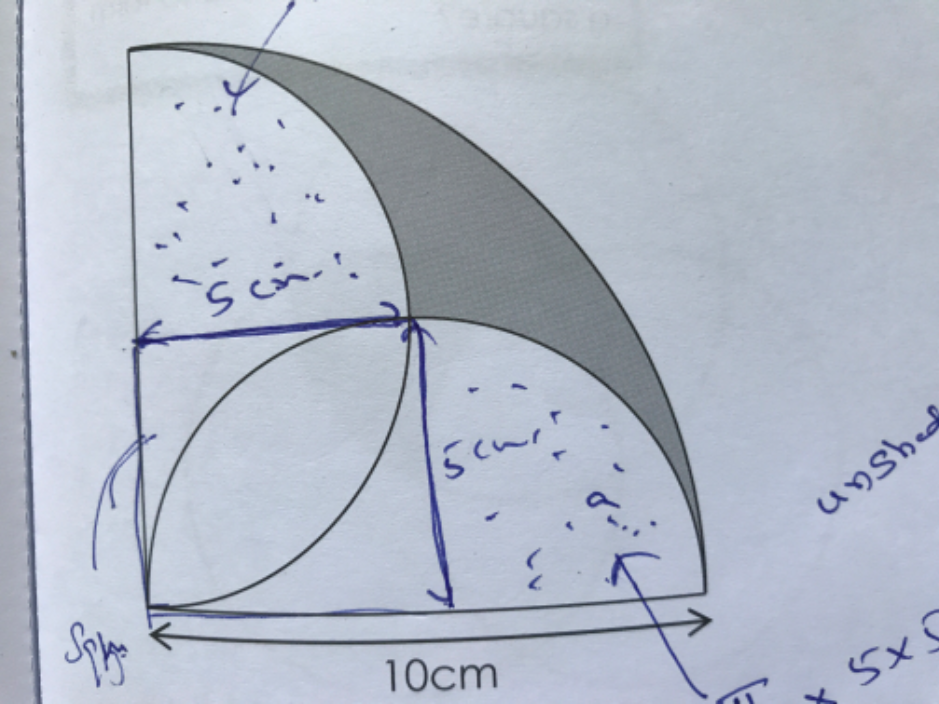# QuestionPicture consists of a circle and two semicircles. Find the area of shaded portion.

Excuse me for the scribble.

big quad= 1/4 x pi x 10 x 10 =25pi

small square= 5 x 5 =25

small quad= 1/4 x pi x 5 x 5 =6.25pi

25pi-(25 + 6.25pi + 6.25pi) = 14.26990817 (round it from here)

0 Replies 0 Likes

Method 1 :
(1/2) x pi x 5 x 5 = 25pi/2
(1/2) x 10 x 5 = 25
area of shaded portion ——- (1/4) x pii x 10 x 10 – (1/2) x 10 x 10 – (25pi/2) + 25 = 14.27

Method 2 :
area of shaded portion ——- (1/4) x pi x 10 x 10  – (1/2) x pi x 5 x 5 – 5 x 5 = 14.27

Ans : 14.27 sq cm

0 Replies 0 Likes

Can try?